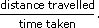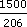Science, Maths & Technology

### Become an OU studentRatio, proportion and percentages

Start this free course now. Just create an account and sign in. Enrol and complete the course for a free statement of participation or digital badge if available.

# 1.5 Speeds

Speed is the ratio of distance travelled to time taken. A runner's speed may be quoted in metres per second, miles per hour or kilometres per hour. The units are given as:

unit of distance per unit of time.

When you have a distance covered (such as a mile) and a time taken (such as four minutes) the average speed is defined as

•The formula for average speed applies even over a journey made up of several stages.

## Example 6

In 1999, Hicham El Guerrouj held the record for running a mile. He covered the distance in just over 3 minutes 43 seconds. In the same year he also held the world record for the 1500 m race. He completed this distance in 3 minutes 26 seconds.

Work out:

• (a) his (average) speed in miles per hour for the 1 mile race;

• (b) his (average) speed in kilometres per hour for the 1500 metre race;

• (c) compare (a) and (b). In which race was his (average) speed faster assuming that 1.61 km = 1 mile?

• (b) Hicham ran 1500 metres in 3 minutes 26 seconds (i.e. 206 seconds), so he ranmetres in 1 second. In 3600 seconds (1 hour) he runs 3600 ×metres. That is, 26 213.59 metres per hour. To convert to kilometres: divide by 1000 which gives 26.21 km per hour (to 2 d.p.).